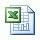# Increase Mud Weight by Adding Calcium Carbonate

Carbonate is weighing agent mostly used in horizontal well because it does not plug up formation. We can use the same weight up concept with barite to calculate how to weight up with carbonate. Follow a topic below to learn how to weight up with calcium carbonate.

NOTE: The maximum practical mud weight attainable with calcium carbonate is 14.0 ppg.

This formula below is used for calculating how many sacks of calcium carbonate required per 100 bbl of drilling fluid (Mud weight increase with calcium carbonate (SG – 2.7))

Sacks of calcium carbonate per 100 bbl of mud = 945 x (W2 – W1) ÷ (22.5 – W2)

Where; W1 = current mud weight in ppg

W2 = new mud weight in ppg

Example: Determine the number of sacks of calcium carbonate per l00 bbl required to increase the density from 10.0 ppg (W1) to 13.0 ppg (W2):
Sacks of calcium carbonate per 100 bbl of mud = 945 x(13.0 – 10.0) ÷ (22.5 – 13.0)

Sacks of calcium carbonate per 100 bbl of mud = 298.4 sacks
If you have total volume of 500 bbl of mud, calcium carbonate required to increase mud weight from 10.0 ppg to 13.0 ppg is 1,492 sacks (298.4×500/100).

Please find theExcel sheet for calculating how many sacks of calcium carbonate required per 100 bbl of drilling fluid.

Share the joy